### Math Monday: Beginning of a String

by Glen Whitney

Although there have been two different Math Mondays touching just tangentially on the wonderful world of string art, it has never covered the basics of the area, and it’s high time. (Why is it high time? Hint: in preparation for non-basics to come…) The fundamental idea is that you create one or more series of connection points, regular in some way, and then stretch taut strings between the connections (again, typically in some orderly fashion), producing (hopefully!) an interesting, beautiful, and/or mathematically significant overall pattern.

The usual starting point is 2n nails driven into a board, at the points (1, 0), (2, 0), (3,0), … , (n-2, 0), (n-1, 0), (n,0) and (0,1), (0,2), … , (0, n-1), (0,n) in some convenient standard coordinate system, like so: (ignore the embroidery floss for the moment)Then you run a string from (1,0) to (0,n), from (2,0) to (0,n-1), from (3, 0) to (0, n-2), and so on, up through a string from (n, 0) to (0, 1). Note that the x coordinate of the start of each segment plus the y coordinate of the end of each segment always adds up to n + 1. Note you don’t have to tie off each segment separately; the most efficient use of string is to tie off at (1,0), run to (0,n), pivot around to (0,n-1) and run from there to (2,0), over to (3,0), back to (0,n-2), down to (0,n-3), back to (4,0), over to (5,0), and so on until the pattern is done. Here’s what it comes out looking like: (It’s a great exercise in intuitive mathematics to guess what curve appears as the envelope of all of the line segments you’ve strung. If you don’t know the answer, try to guess before you scroll down below the picture and read on.)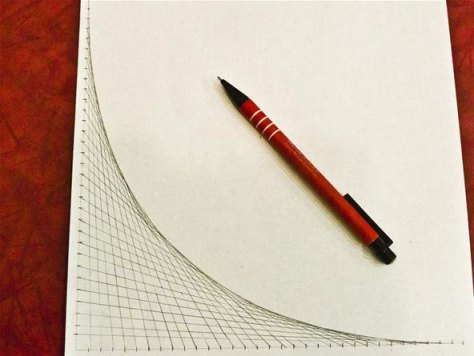To kill some space before the reveal of what curve this is, here are a few variations on this basic stringing technique. Of course, any technique for creating many straight lines will do, such as pulling embroidery floss through holes in cardstock, or drawing many lines with a straightedge, or projecting many laser beams, or whatever else you can come up with…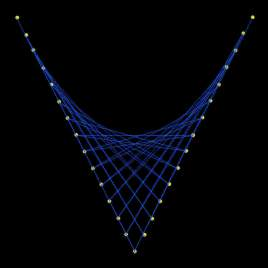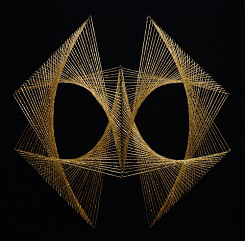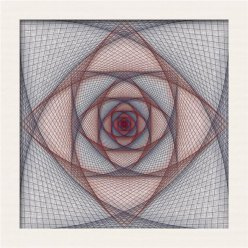OK, here’s the answer to the math question: the envelope of these lines is a parabola (in fact, that’s true of all of the curves in all of the variations above). It’s also a great exercise in formal mathematics to determine the equation for the parabola that all of the lines in the first example shown are tangent to (hint: it’s not of the form y = some second-degree polynomial in x, because that form only gives you parabolas whose axes are vertical lines…), and to prove that they really are tangents.

Of course, it’s possible to get other envelopes of lines if you start with other arrangements of connection points (or for that matter, if you connected the points in a different pattern). For example, with a circle of nails, always stringing a constant number of positions around the circle, you get a star of lines whose envelope is a smaller circle: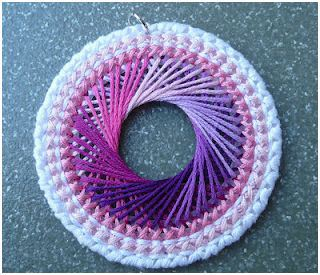Alternatively, if you move one end of the segment a single position around the circle each time but move the other end of the segment two positions, you get a cardiod: (Reader exercise: what is a cardiod? What’s its equation? Why does it show up from this process?)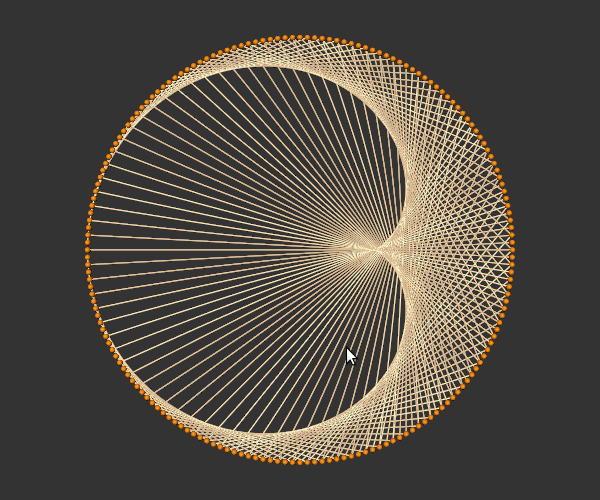So those are the basics of string art. If you’ve got an example of string art of particular mathematical significance, let mondays@momath.org know!